# p-block - JEE Main Previous Year Questions with Solutions

JEE Main Previous Year Papers Questions of Chemistry With Solutions are available at eSaral. Simulator Previous Years AIEEE/JEE Mains Questions
Q. Which one of the following reactions of Xenon compounds is not feasible ? (1) $2 \mathrm{XeF}_{2}+2 \mathrm{H}_{2} \mathrm{O} \rightarrow 2 \mathrm{Xe}+4 \mathrm{HF}+\mathrm{O}_{2}$ (2) $\mathrm{XeF}_{6}+\mathrm{RbF} \rightarrow \mathrm{Rb}\left[\mathrm{XeF}_{7}\right]$ (3) $\mathrm{XeO}_{3}+6 \mathrm{HF} \rightarrow \mathrm{XeF}_{6}+3 \mathrm{H}_{2} \mathrm{O}$ (4) $3 \mathrm{XeF}_{4}+6 \mathrm{H}_{2} \mathrm{O} \rightarrow 2 \mathrm{Xe}+\mathrm{XeO}_{3}+12 \mathrm{HF}+1.5 \mathrm{O}_{2}$ [aieee-2009]
Ans. (3)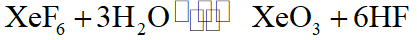Q. Which of the following statement is wrong ? (1) Single N–N bond is weaker than the single P–P bond (2) $\mathrm{N}_{2} \mathrm{O}_{4}$ has two resonance structures (3) The stability of hydrides increases from NH3 to BiH3 in group 15 of the periodic table (4) Nitrogen cannot form $\mathrm{d} \pi-\mathrm{p} \pi$ bond [aieee-2011]
Ans. (3) The stability of hydrides decreases from $\mathrm{NH}_{3}$ to $\mathrm{BiH}_{3}$ in group 15
Q. Which of the following statements regarding sulphur is incorrect ? (1) At $600^{\circ} \mathrm{C}$ the gas mainly consists of $\mathrm{S}_{2}$ molceules (2) The oxidation state of sulphur is never less than +4 in its compounds (3) $\mathrm{S}_{2}$ molecule is paramagnetic (4) The vapour at $200^{\circ} \mathrm{C}$ consists mostly of $\mathrm{S}_{8}$ rings [aieee-2011]
Ans. (2)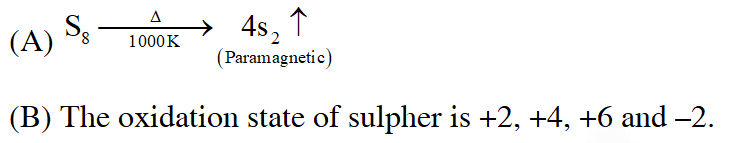Q. Boron cannot form which one of the following anions ? (1) $\mathrm{B}(\mathrm{OH})_{4}^{-}$ (2) $\mathrm{BO}_{2}^{-}$ (3) $\mathrm{BF}_{6}^{3-}$ (4) $\mathrm{BH}_{4}^{-}$ [aieee-2011]
Ans. (3) Due to the absence of vacant $2 \mathrm{d}-$ orbital, $\left[\mathrm{BF}_{6}\right]^{3-}$ is not form
Q. In view of the signs of $\Delta_{\mathrm{r}} \mathrm{G}^{\circ}$ for the following reactions $\mathrm{PbO}_{2}+\mathrm{Pb} \rightarrow 2 \mathrm{PbO}, \Delta_{\mathrm{r}} \mathrm{G}^{\circ}<0$ $\mathrm{SnO}_{2}+\mathrm{Sn} \rightarrow 2 \mathrm{SnO}, \Delta_{\mathrm{r}} \mathrm{G}^{\circ}>0$ Which oxidation states are more characteristic for lead and tin ? (1) For lead + 4, for tin + 2 (2) For lead + 2, for tin + 2 (3) For lead + 4, for tin + 4 (4) For lead + 2, for tin + 4 [aieee-2011]
Ans. (4)
Q. The number of $\mathrm{S}-\mathrm{S}$ bonds in $\mathrm{SO}_{3}, \mathrm{S}_{2} \mathrm{O}_{3}^{2-}, \mathrm{S}_{2} \mathrm{O}_{6}^{2-}$ and $\mathrm{S}_{2} \mathrm{O}_{8}^{2-}$ respectively are :- (1) 1, 0, 1, 0 (2) 0, 1, 1, 0 (3) 1, 0, 0, 1 (4) 0, 1, 0, 1 [JEE(Main)Online-2012]
Ans. (2)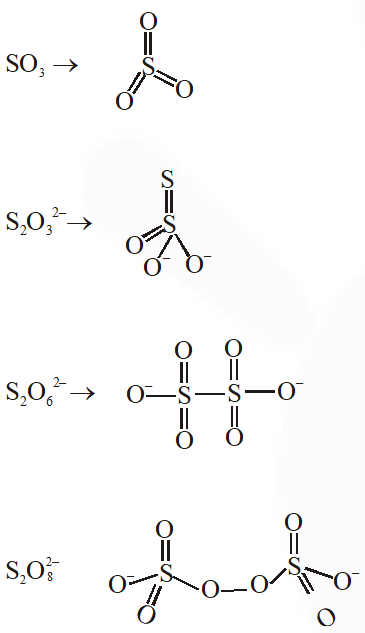Q. Which one of the following depletes ozone layer ? (1) NO and freons (2) $\mathrm{SO}_{2}$ (3) CO ( 4) $\mathrm{CO}_{2}$ [JEE(Main)Online-2012]
Ans. (1)
Q. In which of the following arrangements, the sequence is not strictly according to the property written against it ? (1) $\mathrm{CO}_{2}<\mathrm{SiO}_{2}<\mathrm{SnO}_{2}<\mathrm{PbO}_{2}$ : increasing oxidisin g power (2) B < C < O < N : increasing first ionisation enthalpy (3) $\mathrm{NH}_{3}<\mathrm{PH}_{3}<\mathrm{AsH}_{3}<\mathrm{SbH}_{3}$ : increasing basic strength (4) HF < HCl < HBr < HI : increasing acid strength [JEE(Main)Online-2012]
Ans. (3) Basic strength order : $\mathrm{NH}_{3}>\mathrm{PH}_{3}>\mathrm{AsH}_{3}>\mathrm{SbH}_{3}$
Q. The compound of Xenon with zero dipole moment is :- (1) $\mathrm{XeO}_{3}$ (2) $\mathrm{XeO}_{2}$ (3) $\mathrm{XeF}_{4}$ (4) $\mathrm{XeOF}_{4}$ [JEE(Main)Online-2012]
Ans. (3)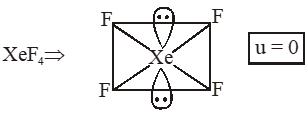Q. Which one of the following depletes ozone layer ? (1) NO and freons (2) $\mathrm{SO}_{2}$ (3) CO (4) $\mathrm{CO}_{2}$ [JEE(Main)Online-2012]
Ans. (1)
Q. The formation of molecular complex $\mathrm{BF}_{3}-\mathrm{NH}_{3}$ results in a change in hybridisation of boron :- (1) from $\operatorname{sp}^{3}$ to $\mathrm{sp}^{3} \mathrm{d}$ (2) from $\mathrm{sp}^{2}$ to $\mathrm{dsp}^{2}$ (3) from $\operatorname{sp}^{3}$ to $\mathrm{sp}^{2}$ (4) from $\mathrm{sp}^{2}$ to $\mathrm{sp}^{3}$ [JEE(Main)Online-2012]
Ans. (4)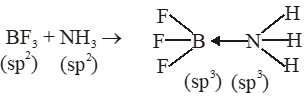Q. Trigonal bipyramidal geometry is shown by: (1) $\mathrm{XeO}_{3} \mathrm{F}_{2}$ (2) $\mathrm{XeOF}_{2}$ (3) $\left[\mathrm{XeF}_{8}\right]^{2-}$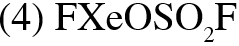[JEE(Main)Online-2013]
Ans. (1)
Q. Oxidation state of sulphur in anions $\mathrm{SO}_{3,}^{2-} \mathrm{S}_{2} \mathrm{O}_{4}^{2-}$ and $\mathrm{S}_{2} \mathrm{O}_{6}^{2-}$ increases in the orders : (1) $\mathrm{S}_{2} \mathrm{O}_{6}^{2-}<\mathrm{S}_{2} \mathrm{O}_{4}^{2-}<\mathrm{SO}_{3}^{2-}$ (2) $\mathrm{SO}_{3}^{2-}<\mathrm{S}_{2} \mathrm{O}_{4}^{2-}<\mathrm{S}_{2} \mathrm{O}_{6}^{2-} (3)$\mathrm{S}_{2} \mathrm{O}_{4}^{2-}<\mathrm{SO}_{3}^{2-}<\mathrm{S}_{2} \mathrm{O}_{6}^{2-}$(4)$\mathrm{S}_{2} \mathrm{O}_{4}^{2-}<\mathrm{S}_{2} \mathrm{O}_{6}^{2-}<\mathrm{SO}_{3}^{2-}$[JEE(Main)Online-2013] Ans. (3)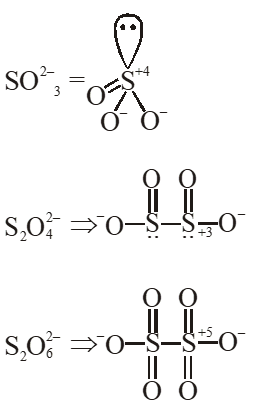Q.$\mathrm{XeO}_{4}$molecule is tetrahedral having: (1) Two p$\pi$- d$\pi$bonds (2) Four p$\pi$- d$\pi$bonds (3) One p$\pi$-d$\pi$bond (4) Three p$\pi$-d$\pi$bonds [JEE(Main)Online-2013] Ans. (2)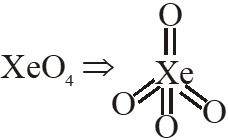$\rightarrow \mathrm{sp}^{3}$hybridisation$\rightarrow$No. of$(\mathrm{p} \pi-\mathrm{d} \pi)$bonds$=4$Q. The shape of \mathrm{IF}_{6}^{-} is : (1) Trigonally distorted octahedron (2) Pyramidal (3) Octahedral (4) Square antiprism [JEE(Main)Online-2013] Ans. (1)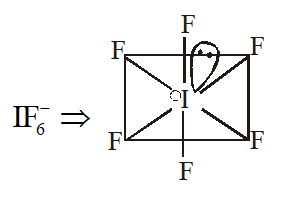Trigonally distorted octahedron Q. Which has trigonal bipyramidal shape ? (1)$\mathrm{XeOF}_{4}$(2)$\mathrm{XeO}_{3}$(3)$\mathrm{XeO}_{3} \mathrm{F}_{2}$(4)$\mathrm{XeOF}_{2}$[JEE(Main)Online-2013] Ans. (3)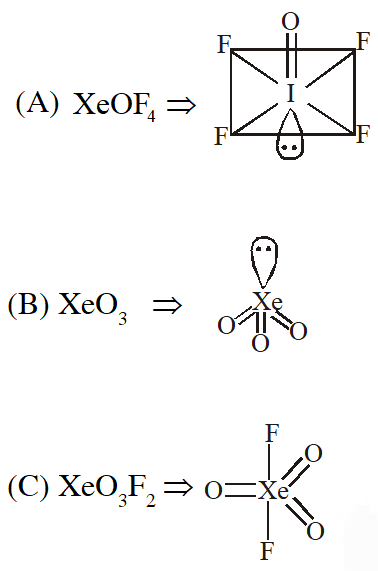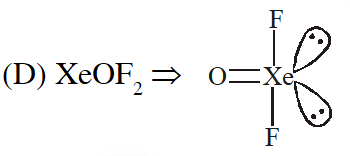Q. The catenation tendency of C, Si and Ge is in the order Ge < Si < C. The bond energies (in kJ$\mathrm{mol}^{-1}$) of C — C, Si —Si and Ge—Ge bonds are respectively : (1) 348, 260, 297 (2) 348, 297, 260 (3) 297, 348, 260 (4) 260, 297, 348 [JEE(Main)Online-2013] Ans. (2) Q. The gas evolved on heating$\mathrm{CaF}_{2}$and$\mathrm{SiO}_{2}$with concentrated$\mathrm{H}_{2} \mathrm{SO}_{4}$, on hydrolysis gives a white gelatinous precipitate. The precipitate is: (1) silica gel (2) silicic acid (3) hydrofluosilicic acid (4) calciumfluorosilicate [JEE(Main)Online-2014] Ans. (2)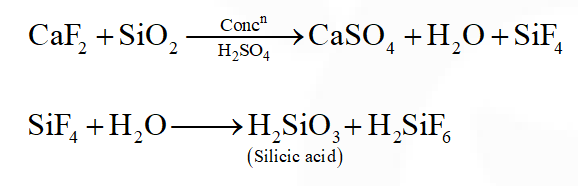Q. Shapes of certain interhalogen compounds are stated below. Which one of them is not correctly stated? (1) IF_$_{7}:$Pentagonal bipyramid (2) BrF$_{5}:$Trigonal bipyramid (3) ICl_3: Planar dimeric (4)$\mathrm{BrF}_{3}:$Planar T-shaped [JEE(Main)Online-2014] Ans. (2)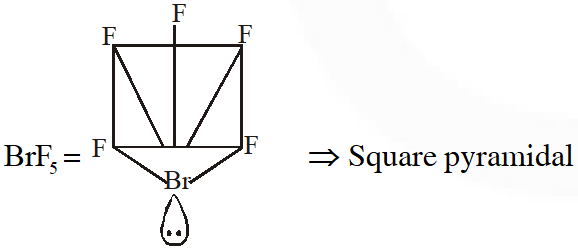Q. Which of the following series correctly represents relations between the elements from X to Y? X  Y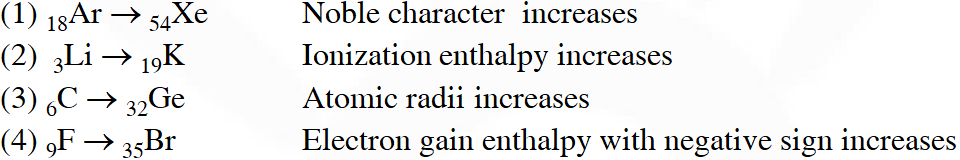[JEE(Main)Online-2014] Ans. (3) Q. Which of the following statements about the depletion of ozone layer is correct? (1) The problem of ozone depletion is more serious at poles because ice crystals in the clouds over poles act as catalyst for photochemical reactions involving the decomposition of ozone by Cl• and ClO• radicals (2) The problem of ozone depletion is less serious at poles because$\mathrm{NO}_{2}$solidifies and is not available for consuming ClO• radicals (3) Oxides of nitrogen also do not react with ozone in stratosphere (4) Freons, chlorofluorocarbons, are inert chemically, they do not react with ozone in stratosphere [JEE(Main)Online-2014] Ans. (1) Q. Which of the following xenon-OXO compounds may not be obtained by hydrolysis of xenon fluorides ? (1)$\mathrm{XeO}_{2} \mathrm{F}_{2}$(2)$\mathrm{XeO}_{3}$(3)$\mathrm{XeO}_{4}$(4)$\mathrm{XeOF}_{4}$[JEE(Main)Online-2014] Ans. (3)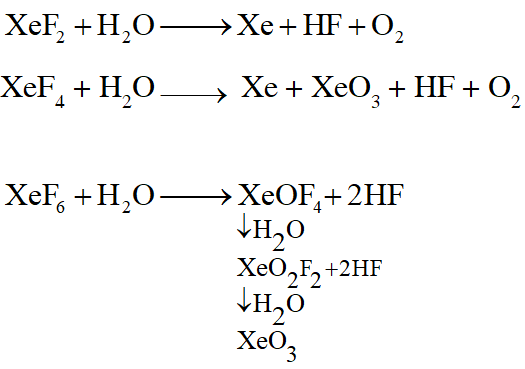Q. Hydrogen peroxide acts both as an oxidising and as a reducing agent depending upon the nature of the reacting species. In which of the following cases$\mathrm{H}_{2} \mathrm{O}_{2}$acts as a reducing agent in acid medium ? (1)$\mathrm{MnO}_{4}^{-}$(2)$\mathrm{SO}_{3}^{2-}$(3) KI (4)$\mathrm{Cr}_{2} \mathrm{O}_{7}^{2-}$[JEE(Main)Online-2014] Ans. (1)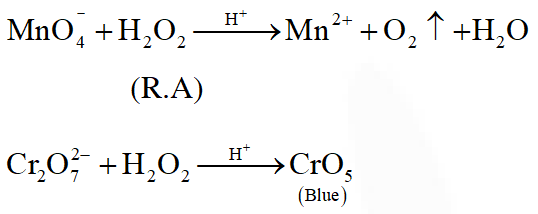Q. Consider the reaction$\mathrm{H}_{2} \mathrm{SO}_{3(\alpha q)}+\mathrm{Sn}_{(\alpha a)}^{4+}+\mathrm{H}_{2} \mathrm{O}_{(1)} \rightarrow \mathrm{Sn}_{(\mathrm{aq})}^{2+}+\mathrm{HSO}_{4(\mathrm{aq})}^{-}+3 \mathrm{H}_{(\mathrm{aq})}^{+}$Which of the following statements is correct? (1)$\mathrm{H}_{2} \mathrm{SO}_{3}$is the reducing agent because it undergoes oxidation (2)$\mathrm{H}_{2} \mathrm{SO}_{3}$is the reducing agent because it undergoes reduction (3)$\mathrm{Sn}^{4+}$is the reducing agent because it undergoes oxidation (4)$\mathrm{Sn}^{4+}$is the oxidizing agent because it undergoes oxidation [JEE(Main)Online-2014] Ans. (1) Q. The number and type of bonds in$\mathrm{C}_{2}^{2-}$ion in$\mathrm{CaC}_{2}$are : (1) Two$\sigma$bonds and one$\pi-$bond (2) Two$\sigma$bonds and two$\pi$- bonds (3) One$\sigma$bond and two$\pi$- bonds (4) One$\sigma$bond and one$\pi$- bond [JEE(Main)Online-2014] Ans. (3)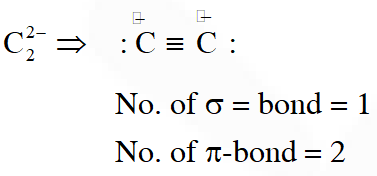Q. In the following sets of reactants which two sets best exhibit the amphoteric character of$\mathrm{Al}_{2} \mathrm{O}_{3} \cdot \mathrm{xH}_{2} \mathrm{O} ?$Set-1$: \mathrm{Al}_{2} \mathrm{O}_{3} \cdot \mathrm{XH}_{2} \mathrm{O}(\mathrm{s})$and$\mathrm{OH}^{-}(\mathrm{aq})$Set$-2: \mathrm{Al}_{2} \mathrm{O}_{3} \cdot \mathrm{XH}_{2} \mathrm{O}(\mathrm{s})$and$\mathrm{H}_{2} \mathrm{O}(\ell)$Set-3$: \mathrm{Al}_{2} \mathrm{O}_{3} \cdot \mathrm{xH}_{2} \mathrm{O}(\mathrm{s})$and$\mathrm{H}^{+}(\mathrm{aq})$Set-4:$\mathrm{Al}_{2} \mathrm{O}_{3} \cdot \mathrm{xH}_{2} \mathrm{O}(\mathrm{s})$and$\mathrm{NH}_{3}(\mathrm{aq})$(1) 1 and 2 (2) 2 and 4 (3) 1 and 3 (4) 3 and 4 [JEE(Main)Online-2014] Ans. (3)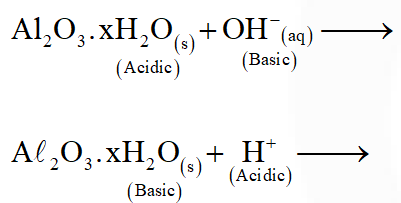Q. Example of a three-dimensional silicate is : (1) Beryls (2) Zeolites (3) Feldspars (4) Ultramarines [JEE(Main)Online-2014] Ans. (1)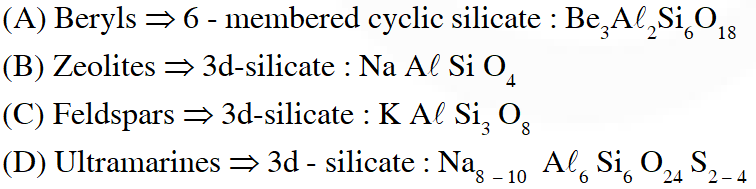Q. Which of the following compounds has a P-P bond :- (1)$\mathrm{H}_{4} \mathrm{P}_{2} \mathrm{O}_{5}$(2)$\left(\mathrm{HPO}_{3}\right)_{3}$(3)$\mathrm{H}_{4} \mathrm{P}_{2} \mathrm{O}_{7}$(4)$\mathrm{H}_{4} \mathrm{P}_{2} \mathrm{O}_{6}$[JEE(Main)Online-2015] Ans. (4)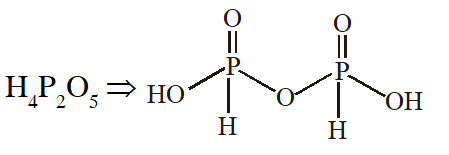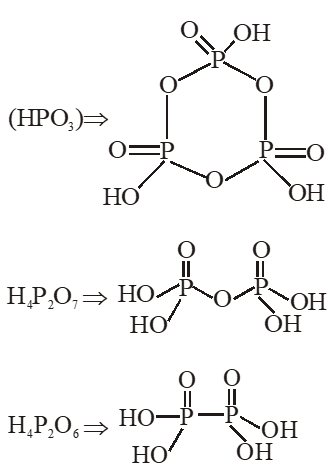Q. Chlorine water on standing loses its colour and forms :- (1)$\mathrm{HCl}$and$\mathrm{HClO}_{2}$(2) HCl only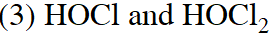(4) HCl and HOCl [JEE(Main)Online-2015] Ans. (4)$\mathrm{C} \ell_{2}+\mathrm{H}_{2} \mathrm{O} \rightarrow \mathrm{HCl}+\mathrm{HOCl}$Q. Which among the following is the most reactive ? (1)$\mathrm{I}_{2}$(2) Icl (3)$\mathrm{Cl}_{2}$( 4)$\mathrm{Br}_{2}$[JEE-Main2015] Ans. (2) Inter halogen compounds are more reactive than halogen except$\mathrm{F}_{2}$. Q. Which one has the highest boiling point ? (1) Kr (2) Xe (3) He (4) Ne [JEE-Main2015] Ans. (2) Boiling point order: He < Ne < Ar < Kr < Xe Q. From the following statements regarding$\mathrm{H}_{2} \mathrm{O}_{2}$, choose the incorrect statement : (1) It has to be stored in plastic or wax lined glass bottles in dark (2) It has to be kept away from dust (3) It can act only as an oxidizing agent (4) It decomposes on exposure to light [JEE-Main2015] Ans. (3)$\mathrm{H}_{2} \mathrm{O}_{2}$acts as aciding as well as reducing nature.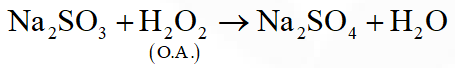Q. Which of the following are Lewis acids ? (1)$\mathrm{AlCl}_{3}$and$\mathrm{SiCl}_{4}$(2)$\mathrm{PH}_{3}$and$\mathrm{SiCl}_{4}$(3)$\mathrm{BCl}_{3}$and$\mathrm{AlCl}_{3}$(4)$\mathrm{PH}_{3}$and$\mathrm{BCl}_{3}$[JEE-Main2018] Ans. (3)$\mathrm{BCl}_{3} \& \mathrm{AlCl}_{3}$both have vacant p-orbital \& incomplete octet. So they act as Lewis acid. Q. The compound that does not produce nitrogen gas by the thermal decomposition is ( 1)$\left(\mathrm{NH}_{4}\right)_{2} \mathrm{Cr}_{2} \mathrm{O}_{7}$(2)$\mathrm{NH}_{4} \mathrm{NO}_{2}$(3)$\left(\mathrm{NH}_{4}\right)_{2} \mathrm{SO}_{4}$(4)$\mathrm{Ba}\left(\mathrm{N}_{3}\right)_{2}\$ [JEE-Main2018]
Ans. (3)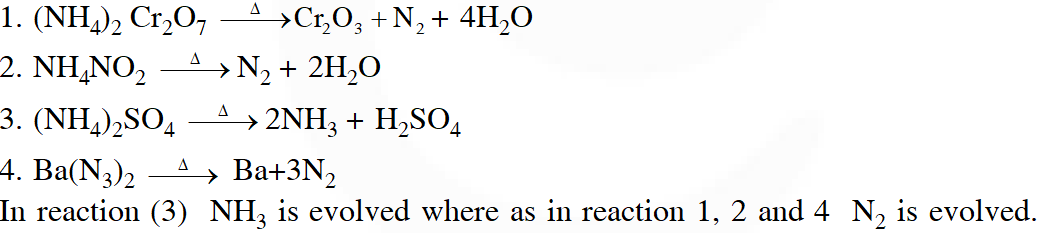aleva strom
Feb. 26, 2022, 10:16 p.m.
it is good to study like this i need more questions the most recent years
rock
Aug. 28, 2021, 6:59 a.m.
all three are three dimension species beryls zeolits faldspare ultramarines
Fhfh
March 5, 2021, 3:21 p.m.
Eska PDF kaha milega
Purush darling
Feb. 22, 2021, 6:18 a.m.
February 2021 syallabus it's enough
sweety
Feb. 8, 2021, 3:32 p.m.
good
Nit ian
Jan. 28, 2021, 9:53 p.m.
ONE OF THE BEST ❤️👌 👌
Mr. T
Dec. 29, 2020, 1:29 p.m.
Thank you
Priyanshu
Dec. 3, 2020, 2:20 p.m.
Great
sashavali
Nov. 14, 2020, 1:02 p.m.
please provide 2019 and 2020 questions also
Nishant
Nov. 8, 2020, 10:03 p.m.
I love you esaraal
Malia talwar
Nov. 6, 2020, 11:53 p.m.
Good enough
sjdckdc
Sept. 26, 2020, 6:04 a.m.
excellent
Parshant
Sept. 25, 2020, 12:46 a.m.
Really helpful Thanks you so much for this
Bhumika mittal
Sept. 21, 2020, 4:50 p.m.
Photos are not available why?
Abhishek
Sept. 6, 2020, 10:33 a.m.
I didn't get all the questions. 😔
Vaidehi
Sept. 5, 2020, 9:23 a.m.
Best notes for preparation.
sweety
Sept. 4, 2020, 6:04 p.m.
tQ SOOO much it is very helpful
Sanket
Sept. 1, 2020, 9:29 a.m.
The question are very useful in jee aspirants
Miraal
Aug. 30, 2020, 7:56 p.m.
These questions are helpful for neet as well as jee aspirants
RISHI
Aug. 30, 2020, 5:54 p.m.
we want recent year papers also ..................
Vikas kushwaha
Aug. 29, 2020, 11:04 p.m.
Srigowthami
Aug. 27, 2020, 5:06 p.m.
Thanks ..these qns helped me a lot ..for preparation of grand tests.... Once again thank you so much 🤗🤗
Shivani
Aug. 26, 2020, 10:59 p.m.
Nyc questions it was very useful......
myth
Aug. 26, 2020, 11:45 a.m.
these questions are soo good they help me for my grand test preparation
Poorvi
Aug. 23, 2020, 3:22 p.m.
Nice questions hope these r very useful for my coming xams
Tabasum
Aug. 20, 2020, 7:37 p.m.
Very useful 💯tq
Tabasum
Aug. 14, 2020, 8:57 p.m.
Thanku
Rishi
Aug. 12, 2020, 9:17 a.m.
These que really helped me. Thank you
Hemant thakur
Aug. 9, 2020, 8:04 p.m.
Good question based on jee
susmitha
Aug. 5, 2020, 3:21 p.m.
The questions are really excellent. These questions also describes to be careful with the particular topics.kindly post 2020 questions
Shruti
Aug. 1, 2020, 2:19 p.m.
Best platform
Deves
July 20, 2020, 1:18 a.m.
It really help me a lot....These are such a interesting question that I totally forgot about time....And I spend 2 hours by learning all the concepts please update itt with new problems
Sohom
July 12, 2020, 8:43 a.m.
It really helped me a lot esaral kindly update the questions to 2020.Thank you.
Siddharth
June 22, 2020, 9:36 a.m.
This questions help us to revise whole chapter in just 15 minutes.... Thank you so much
Shiva
June 21, 2020, 10:14 p.m.
Tq
Vivek Mohan J
June 5, 2020, 10:33 p.m.
Thanks a lot it was very helpful
Rishi
June 5, 2020, 6:55 p.m.
Tssm
Kavya
June 4, 2020, 1:35 p.m.
thank you
Knight rider
May 30, 2020, 9:09 a.m.
Tqsm but what about 16 and 17 years there is no Q from p block in these years
Nagasri Jampula
May 9, 2020, 11 p.m.
Tqsm
Aryan Mishra
May 9, 2020, 3:56 p.m.
Very nice thank you
Ayush
April 22, 2020, 8:04 p.m.
Thank you so much
Abhishek.
April 9, 2020, 12:28 a.m.